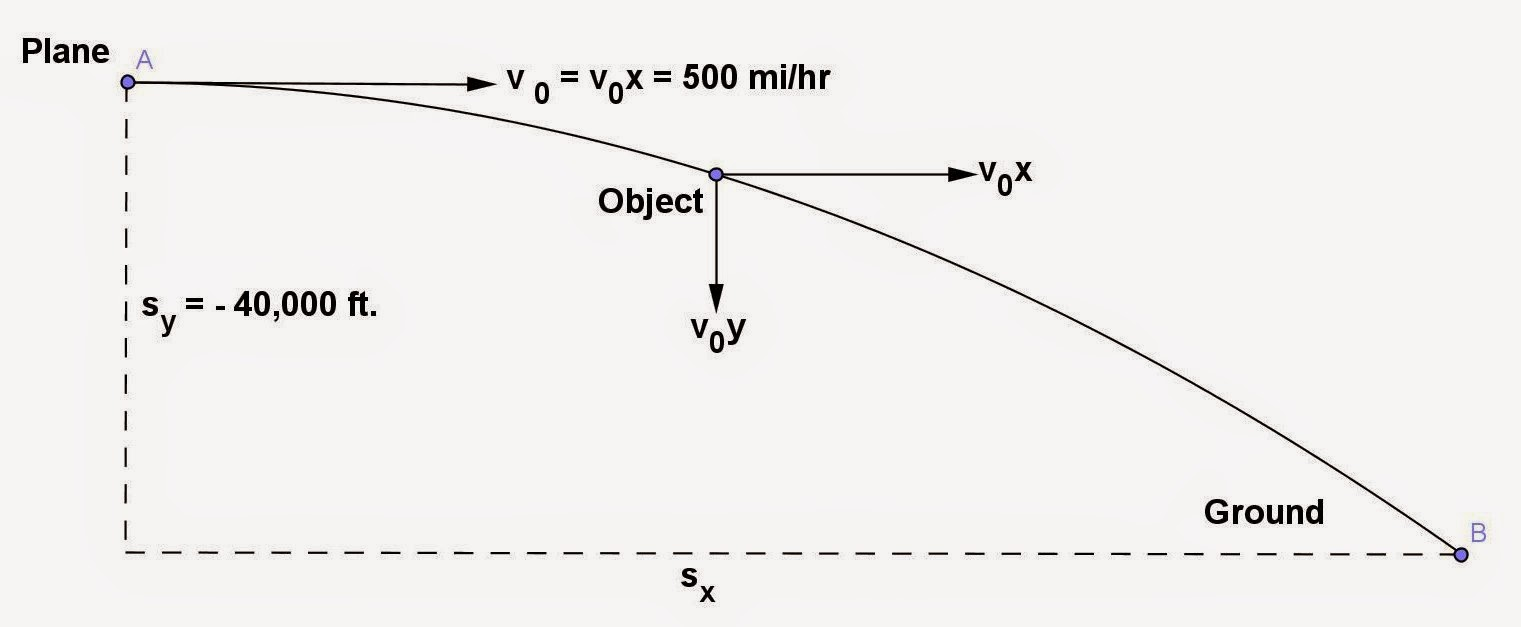Showing posts with label Mechanics. Show all posts
Showing posts with label Mechanics. Show all posts

## Tuesday, November 18, 2014

### Rate, Distance, and Time Problems, 9

Category: Mechanics, Physics

"Published in Newark, California, USA"

Felix rode 18 miles downstream and back to the same place where he started, in 3 hours and 12 minutes. Find the rate of Felix in still water if the velocity of the stream is 3 miles per hour.

Solution:

To illustrate the problem, it is better to draw the figure as followsPhoto by Math Principles in Everyday Life

Let s = be the distance traveled by Felix in upstream / downstream
v = be the velocity or speed of Felix in still water
v1 = be the velocity in downstream
v2 = be the velocity in upstream
t1 = time traveled in downstream
t2 = time traveled in upstream

If the word statement says "Felix rode 18 miles downstream and back to the same place where he started, in 3 hours and 12 minutes.", then the working equation is

Substitute the values of t1 and t2 so that we can solve for the value of v which is Felix' speed or velocity in still water.

By using quadratic formula, the value of v is

We need to choose the positive sign not the negative sign because the value of velocity will be negative which is not accepted as a final answer.  Therefore, the velocity or speed of Felix in still water is

## Monday, October 20, 2014

### Projectile Motion Problems

Category: Mechanics, Physics

"Published in Newark, California, USA"

An object falls from a plane flying horizontally at an altitude of 40,000 ft. at 500 mi/hr. How long will it take to hit the ground? What is the horizontal distance traveled of an object?

Solution:

The given problem is about projectile motion in which an object is thrown from its maximum height with an initial velocity. The projectile curve is a half-parabola as shown in the figure belowPhoto by Math Principles in Everyday Life

First, let's consider the y-component of an object in order to calculate the time required to hit the ground. From the distance formula

Since an object is thrown in a downward position, then the values of sy and ay are negative. ay is also equal to g = 32.174 ft/sec²  which is an acceleration due to gravity. V0y is zero because the direction of initial velocity of an object is horizontal. Therefore, the time required of an object to hit the ground is

Next, let's consider the x-component of an object in order to calculate the horizontal distance traveled of an object. From the distance formula

Since the direction of v0x is horizontal and constant, then ax is equal to zero. Therefore, the horizontal distance traveled of an object is

or

## Sunday, October 19, 2014

### Rate, Distance, and Time Problems, 8

Category: Physics, Mechanics

"Published in Newark, California, USA"

A runner A can run the mile race in 4.25 min. Another runner B requires 4.55 min to run this distance. If they start out together and maintain their normal speeds, how far apart will they be at the finish of the race?

Solution:

The given problem is about rate, distance, and time problem in which the two runners run in the same mile race. From the given problem also, runner A is faster than runner B because runner A has a lesser time to complete the mile race than runner B.

The speed of runner A is

The speed of runner B is

If the two runners will start together in a mile race, then runner A will finish the race. Therefore, their distance between the two runners at the end of the race is

For runner B, we use the time for runner A because runner A will finish the race.

or